Question

Beryllium-8 is an unstable isotope and decays into two α particles, which are helium nuclei with mass 6.68×10−27kg﻿. This decay process releases 1.5×10−14J﻿ of energy. For this problem, let's assume that the mass of the Beryllium-8 nucleus is just twice the mass of an α particle and that all the energy released in the decay becomes kinetic energy of the α particles. If the Beryllium-8 nucleus is moving in the positive x-direction with a speed of 1.0×106 m/s when it decays, what is the speed of the slower-moving α particle after it is released? Assume that the α particles move entirely in the x-direction.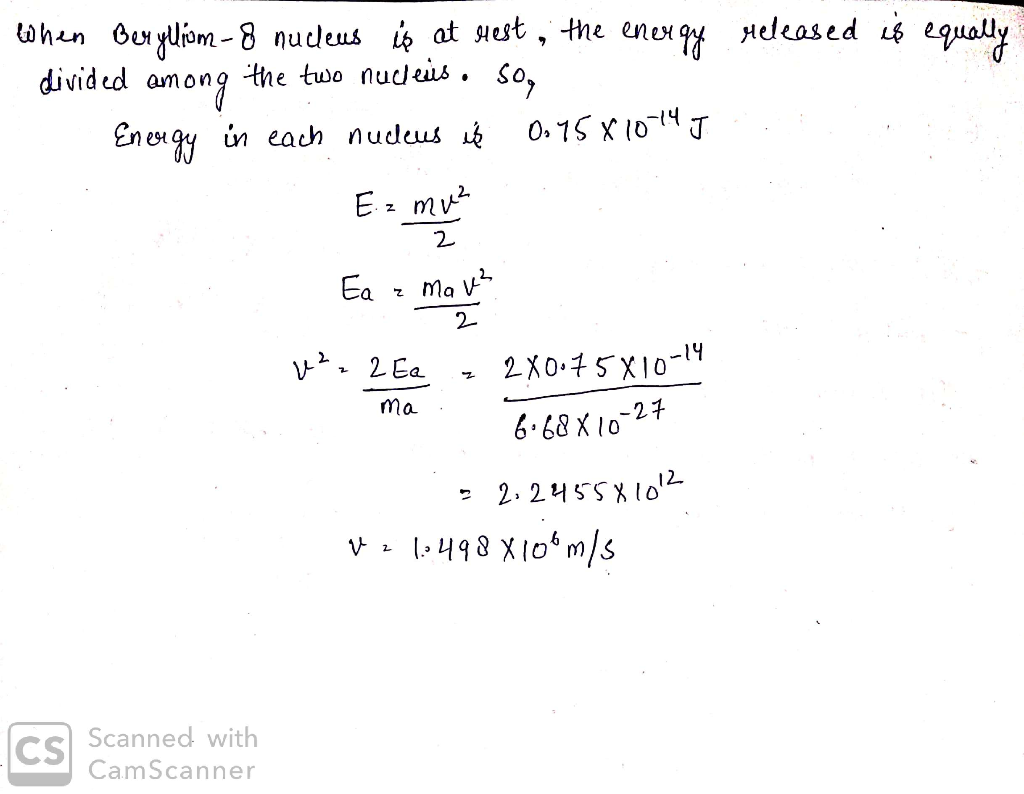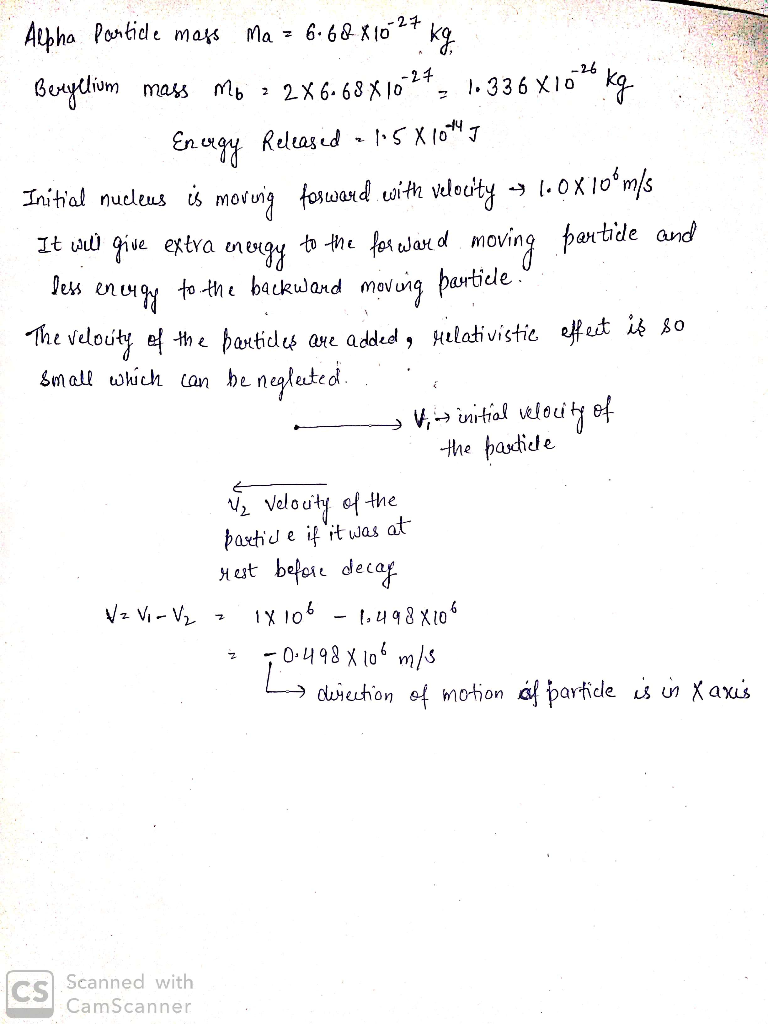#### Earn Coins

Coins can be redeemed for fabulous gifts.

Similar Homework Help Questions
• ### The nucleus of 8Be, which consists of 4 protons and 4 neutrons, is very unstable and spontaneously breaks into two alpha particles (helium nuclei, each with two protons and two neutrons)

The nucleus of 8Be, which consists of 4 protons and 4 neutrons, is very unstable and spontaneously breaks into two alpha particles (helium nuclei, each with two protons and two neutrons). What is the magnitude of the force between the two alpha particles when they are 5 fm (femtometers) apart? What is the magnitude of the acceleration each particle experiences. Take the mass of the alpha particle to be roughly 4 times the proton mass.I need the solutions in as...

• ### A beam of α particles (helium nuclei) is used totreat a tumor located 10 cnn inside...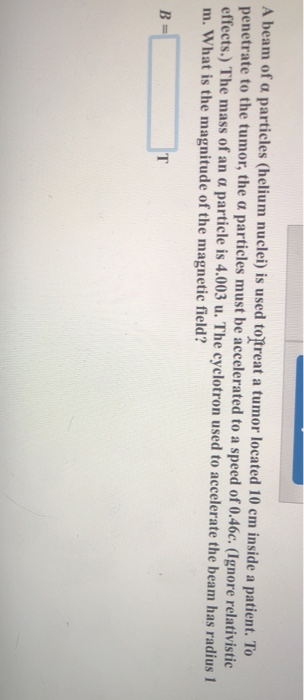A beam of α particles (helium nuclei) is used totreat a tumor located 10 cnn inside a patient. "To penetrate to the tumor, the particles must be accelerated to a speed of 0.46c. (Ignore relativistic effects.) The mass of an a particle is 4.003 u. The cyclotron used to accelerat m. What is the magnitude of the magnetic field? e the beam has radius 1

• ### Use the following information to answer this analytic question The sun produces energy through nuclear fusion....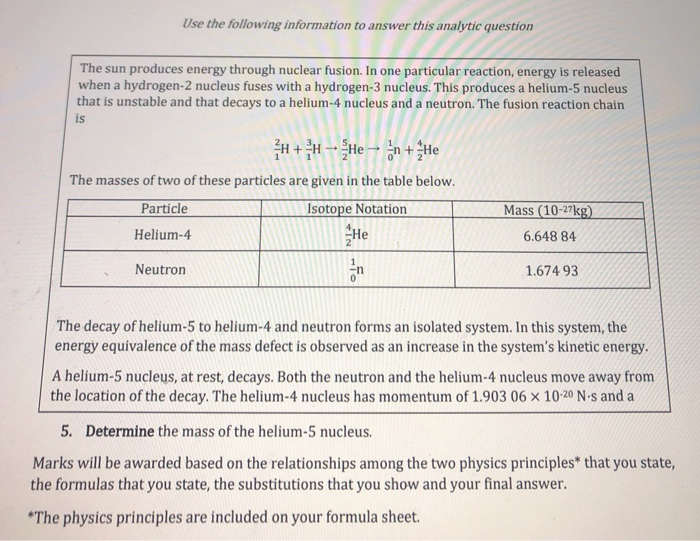Use the following information to answer this analytic question The sun produces energy through nuclear fusion. In one particular reaction, energy is released when a hydrogen-2 nucleus fuses with a hydrogen-3 nucleus. This produces a helium-5 nucleus that is unstable and that decays to a helium-4 nucleus and a neutron. The fusion reaction chain is H + > - He – În + He The masses of two of these particles are given in the table below. Particle Isotope Notation...

• ### Ernest Rutherford (the first New Zealander to be awarded the Nobel Prize in chemistry) demonstrated that nuclei wer...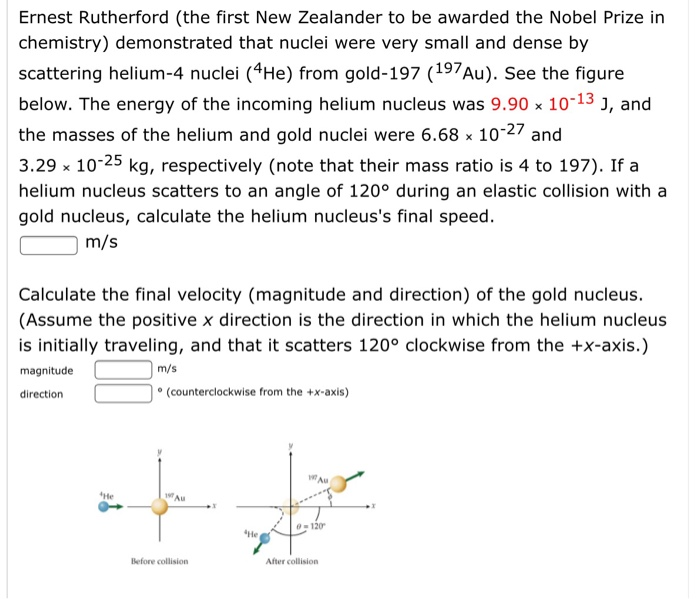Ernest Rutherford (the first New Zealander to be awarded the Nobel Prize in chemistry) demonstrated that nuclei were very small and dense by scattering helium-4 nuclei (4He) from gold-197 (197 Au). See the figure below. The energy of the incoming helium nucleus was 9.90 x 10-13 J, and the masses of the helium and gold nuclei were 6.68 x 10-27 and 3.29 x 10-25 kg, respectively (note that their mass ratio is 4 to 197). If a helium nucleus scatters...

• ### Alpha decay is the emission of alpha particles which are helium nuclei (each contains 2 protons...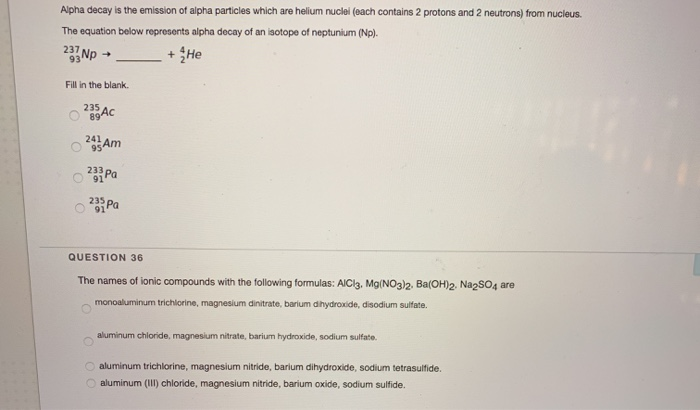Alpha decay is the emission of alpha particles which are helium nuclei (each contains 2 protons and 2 neutrons) from nucleus. The equation below represents alpha decay of an isotope of neptunium (Np). 233 Np + + He Fill in the blank o 225Ac 245 AM 235 Pa QUESTION 36 The names of ionic compounds with the following formulas: AlCl3, Mg(NO3)2, Ba(OH)2. Na2SO4 are monoaluminum trichlorine, magnesium dinitrate, barium dihydroxide, disodium sulfate. aluminum chloride, magnesium nitrate, barium hydroxide, sodium sulfate...

• ### An unstable atomic nucleus of mass 1.50 10-26 kg initially at rest disintegrates into three particles....

An unstable atomic nucleus of mass 1.50 10-26 kg initially at rest disintegrates into three particles. One of the particles, of mass 5.12 10-27 kg, moves in the y direction with a speed of 6.00 106 m/s. Another particle, of mass 8.44 10-27 kg, moves in the x direction with a speed of 4.00 106 m/s. (a) Find the velocity of the third particle. (b) Find the total kinetic energy increase in the process.

• ### An atom of beryllium (m 8.00 u) splits into two atoms of helium (m-4.00 u) with...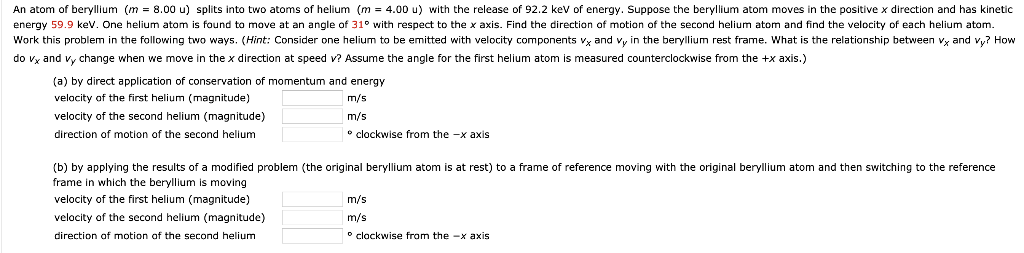An atom of beryllium (m 8.00 u) splits into two atoms of helium (m-4.00 u) with the release of 92.2 keV of energy. Suppose the beryllium atom moves in the positive x direction and has kinetic energy 59.9 keV. One helium atom is found to move at an angle of 31° with respect to the x axis. Find the direction of motion of the second helium atom and find the velocity of each helium atom Work this problem in the...

• ### An unstable atomic nucleus of mass 1.58 10-26 kg initially at rest disintegrates into three particles. One of the parti...

An unstable atomic nucleus of mass 1.58 10-26 kg initially at rest disintegrates into three particles. One of the particles, of mass 5.20 10-27 kg, moves in the y direction with a speed of 6.00 106 m/s. Another particle, of mass 8.44 10-27 kg, moves in the x direction with a speed of 4.00 106 m/s. (a) Find the velocity of the third particle. i + j m/s (b) Find the total kinetic energy increase in the process. J Please...

• ### In 1911, Ernest Rutherford and his assistants Geiger and Marsden conducted an experiment in which they...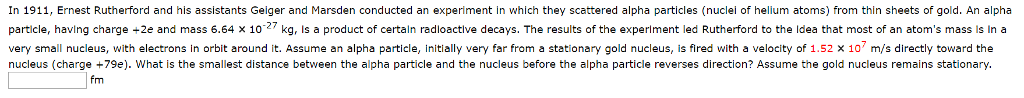In 1911, Ernest Rutherford and his assistants Geiger and Marsden conducted an experiment in which they scattered alpha particles (nuclei of helium atoms) from thin sheets of gold. An alpha particle, having charge ÷2e and mass 6.64 × 10-27 kg, is a product of certain radioactive decays. The results of the experiment led Rutherford to the idea that most of an atom's mass is In a very small nucleus, with electrons in orbit around it. Assume an alpha particle, initially...

• ### In 1911, Ernest Rutherford and his assistants Geiger and Marsden conducted an experiment in which they...

In 1911, Ernest Rutherford and his assistants Geiger and Marsden conducted an experiment in which they scattered alpha particles (nuclei of helium atoms) from thin sheets of gold. An alpha particle, having charge +2e and mass 6.64 10-27 kg, is a product of certain radioactive decays. The results of the experiment led Rutherford to the idea that most of an atom's mass is in a very small nucleus, with electrons in orbit around it. Assume an alpha particle, initially very...